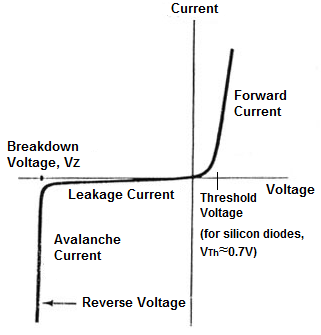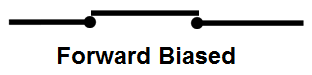﻿ What is an Ideal Diode? ﻿# What is an Ideal Diode?An ideal diode is a diode that acts like a perfect conductor when voltage is applied forward biased and like a perfect insulator when voltage is applied reverse biased.

So when positive voltage is applied across the anode to the cathode, the diode conducts forward current instantly. When voltage is applied in reverse, the diode conducts no current at all.

Below is a graph of the I-V characteristics curve of an ideal diode:

### Ideal Diode Characteristics CurveYou can see that when the diode receives forward voltage, it instantly conducts an infinite amount of current which it can supply to a circuit. When reverse voltage is fed to the diode, it conducts no current at all, no matter how great this reverse voltage is.

These, again, are ideal circumstances. Diodes actually do not act like this.

The I-V characteristics curve of a conventional diode would look like the following shown below.

### Conventional Diode Characteristics CurveBelow is a chart showing the differences between ideal diodes and conventional diodes:

 Characteristics Ideal Diode Coventional Diode Threshold Voltage Ideal diodes do not have a threshold voltage. Once any forward voltage is applied across the diode, it will conduct current instantly across its junctions. Conventional diodes do have a threshold voltage. This is the voltage which must be supplied to the diode for it to conduct any considerable forward current. For silicon diodes, the threshold voltage is approximately 0.7V. For germanium diodes, it is approximately 0.3V. This voltage is needed so that the electrons of the n junction can have enough push to break the barrier in between and cross over into the p junction. This is how current flows in a diode. Any voltage below the threshold voltage will not be sufficient to push the electrons through from one barrier to the next. You can see this marked on the diode characteristics curve. Before the threshold point, very little current flows across the diode. However, after the diode receives a voltage above this, it produces considerable current flow. Forward Current Ideal diodes have infinite forward current when any forward voltage is applied across their terminals. This is because in the ideal condition, the internal resistance of the diode would be 0. The diode would have no internal resistance at all. Since current, I=V/R, an infinite amount of current would be conducted and supplied to a circuit with an ideal diode. Conventional diodes conduct a large current when forward voltage above its threshold voltage is supplied to the diode, but it is still a finite amount of current. Conventional diodes, even though small, still have internal resistance. It is impossible to create any physical component that does not have some internal resistance. The resistance ensures that the current will be finite in nature and cannot be infinite. Breakdown Voltage Ideal diodes do not have a breakdown voltage. This is because ideal diodes have infinite resistance to reverse voltage. It will not conduct any current at all when voltage is applied in reverse, no matter how great the voltage is. Conventional diodes do have a breakdown voltage. This is the reverse voltage that when applied to the diode will cause the diode junctions to break down and conduct a large amount of current, even though the voltage is applied with incorrect polarity. A diode should not conduct current when voltage is applied the wrong way. However, after a certain point, called the breakdown point, it will give way and conduct. Reverse (leakage) Current Since an ideal diode does not have a breakdown point,  it never conducts any reverse current, called leakage current. It is a perfect insulator when voltage is applied in reverse. A conventional diode will conduct some leakage current even when reverse voltage is applied, even when the reverse voltage hasn't reach the breakdown point. After the breakdown voltage is reached, it will conduct a large amount of current, called avalanche current, in reverse. This is because a conventional diode can never be a perfect insulator and will give way if enough reverse voltage is supplied to it.

Like stated above, an ideal diode is impossible to produce. Diodes have a junction barrier they must overcome, so they need a threshold voltage. Diodes cannot conduct infinite current because they will always have some internal resistance. And diodes cannot be perfect insulators when reverse biased, so they will conduct some leakage current in reverse.

The closest real-world item that an ideal diode can be compared to or explained in terms of is a simple SPST switch.

When forward biased, the ideal diode acts like a closed switch:When reverse biased, the ideal diode acts like an open switch.The ideal diode, therefore, is a semiconductor device that is not a switch, but functions as a perfect, intelligent switch which knows when to shut off and turn on based on the voltage applied to its junctions.

So why even study ideal diodes or any ideal components, since they do not exist? The answer is, it helps us learn more about how diodes function if they had perfect conditions. Therefore, it helps us to better understand the characteristics of a diode in the simplest approach. How would a diode act if it didn't have any internal resistance? Therefore, when we know add these elements, we better understand the actual diode curve. So in a way, it provides an excellent starting point for understanding diodes in their simplest (ideal) state. We then can move on to actual real-life characteristics with a better understanding.

Related Resources